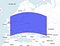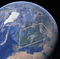Squaring the sphere

Let’s talk about geographical bounding boxes and how they differ from geometric ones.

Bounding boxes are easy when you are on a 2D plane. E.g. PostGIS has a box2d type that describes rectangular geometry. It is usually described by low-left and up-right points. You can ask for geometry’s bounding box, or check if a geometry intersects some box, by implicitly using box2d type as geometry:

-- PostgreSQL code, don't try in BigQuery
SELECT ST_Intersects(ST_Point(2, 3),
ST_MakeBox2D(ST_Point(1, 2), ST_Point(3, 4)))

Things are different in BigQuery or in PostgreSQL when you use Geography type. The Earth is not flat, and the Geography type works on a sphere. What corresponds to the bounding box on the sphere? If we take a “rectangle” Geography shape, say POLYGON((0 40, 60 40, 60 60, 0 60, 0 40)) it will look nothing like a rectangle since Geography uses geodesic edges. Here is how it looks on a flat map:POLYGON((0 40, 60 40, 60 60, 0 60, 0 40)) on flat map

And it is not much of a rectangle on the sphere either:POLYGON((0 40, 60 40, 60 60, 0 60, 0 40)) on the globe

OK, the geodesic edges don’t make much sense for a rectangular bounding box. Let’s switch back to the planar map, and use its Cartesian edges. We’ll have a true rectangle on the map, and it is most useful when filtering objects for showing on such a planar map. The planar box looks like this on the globe:

Unfortunately, the resulting shape can no longer be described by a Geography object in BigQuery, as we would need CurvePolygon geometry type to describe those, which is not supported. The best we can do is approximate the two parallels with a lot of smaller geodesic edges that follow the parallels. BigQuery does this when we read a GeoJson geometry describing this rectangle. But the result could be a complex shape, making it useless for any practical usage. Instead, BigQuery has ST_IntersectsBox function that accepts left (Westmost), low (Southmost), right (Eastmost), and high (Northmost) coordinates, like

-- BigQuery version of the query
SELECT ST_IntersectsBox(ST_GeogPoint(2, 3), 1, 2, 3, 4)

OK, we’ve figured out what’s going on with latitudes and parallels, but what about longitudes? They are usually simple, but let’s see how one would describe the bounding box of Alaska. Wikipedia says its longitudes span from 130°W to 172°E. But if we start from 130°W (Eastmost end of Alaska) and move West until we reach 172°E, we would cover most of the globe, except Alaska. We should describe it as a span from 172°E to 130°W, or from 172 to -130, and we can check if a point intersects the Alaska bounding box using the expression

ST_IntersectsBox(geo, 172, 51, -130, 72)

Note that our box looks strange — min_x coordinate (172º) is greater than max_x (-130º). But that’s OK — remember that the min_x is the Westmost longitude, and max_x is Eastmost, so we are moving from 172º, cross antimeridian, and arrive at -130º. But to restore common sense and make min_x less than max_x, you can wrap the rectangle and use the following expression that results in the same answer:

--  (172 - 360) == -188
ST_IntersectsBox(geo, -188, 51, -130, 72)

or

--  (-130 + 360) == 230
ST_IntersectsBox(geo, 172, 51, 230, 72)

All three versions describe the same bounding box:

However, don’t go too far, BigQuery still checks the bounding box is reasonable, and max_x — min_x < 360. E.g. BigQuery does not accept a bounding box [172, 51, 580, 72]: I’ve added 720 here to the second longitude, and now the semantics of the box becomes confusing — does it wrap around the globe? BigQuery rejects it for this reason.

Finally, if you do want to describe a band between two latitudes that wraps around the whole globe, the way to do it is bypassing longitudes -180 and 180 to ST_IntersectsBox: ST_IntersectsBox(geo, -180, lat1, 180, lat2).

Geospatial Analytics

Codes, maps, ideas, and tutorials made by Geospatial experts

Written by

Michael Entin

Hi, I'm TL of BigQuery GIS project. Posting small recipes and various notes for BQ GIS users.Geospatial Analytics

Codes, maps, ideas, and tutorials made by Geospatial experts